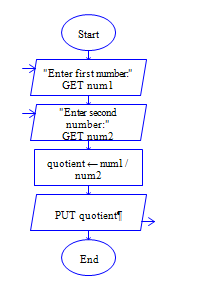# Java Program to divide two numbers

Get two integer numbers, divide both the integers and display the quotient.

Sample Input 1:

6 5

Sample Output 1:

1

Sample Input 2:

28 4

Sample Output 2:

7

#### Flow Chart Design#### Program or Solution

``` import java.util.*; class Division { public static void main(String args[]) { int Input1,Input2,div; Scanner sc=new Scanner(System.in); System.out.println("Enter The Input 1:"); Input1=sc.nextInt(); System.out.println("Enter The Input 2:"); Input2=sc.nextInt(); div=Input1/Input2; System.out.println("The Output Is:\n"+div); } } ```

#### Program Explanation

Get two integers Input1 and Input2 (using scanner class) Divide Input1 by Input2, then store quotient in div (div=Input1/Input2, Note "/" operator gives quotient) Print the value of div (using system.out.println)